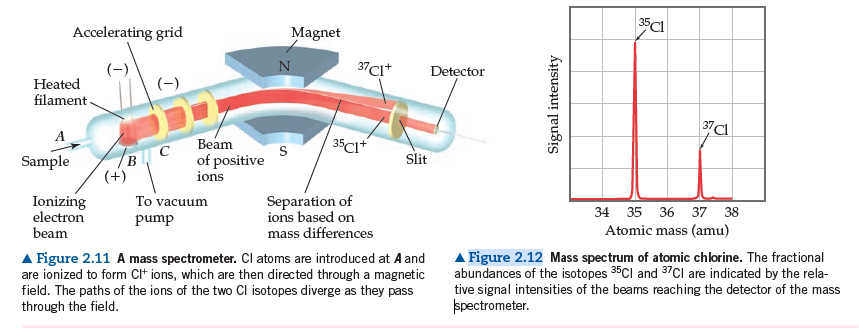# Problem: The mass spectrometer in Figure 2.11 in the textbook has a magnet as one of its components.What is the purpose of the magnet?

###### FREE Expert Solution

We are asked what is the purpose of the magnet.

90% (336 ratings)###### Problem Details

The mass spectrometer in Figure 2.11 in the textbook has a magnet as one of its components.What is the purpose of the magnet?

Frequently Asked Questions

What scientific concept do you need to know in order to solve this problem?

Our tutors have indicated that to solve this problem you will need to apply the Mass Spectrometry concept. If you need more Mass Spectrometry practice, you can also practice Mass Spectrometry practice problems.

What textbook is this problem found in?

Our data indicates that this problem or a close variation was asked in Chemistry: The Central Science - Brown 13th Edition. You can also practice Chemistry: The Central Science - Brown 13th Edition practice problems.